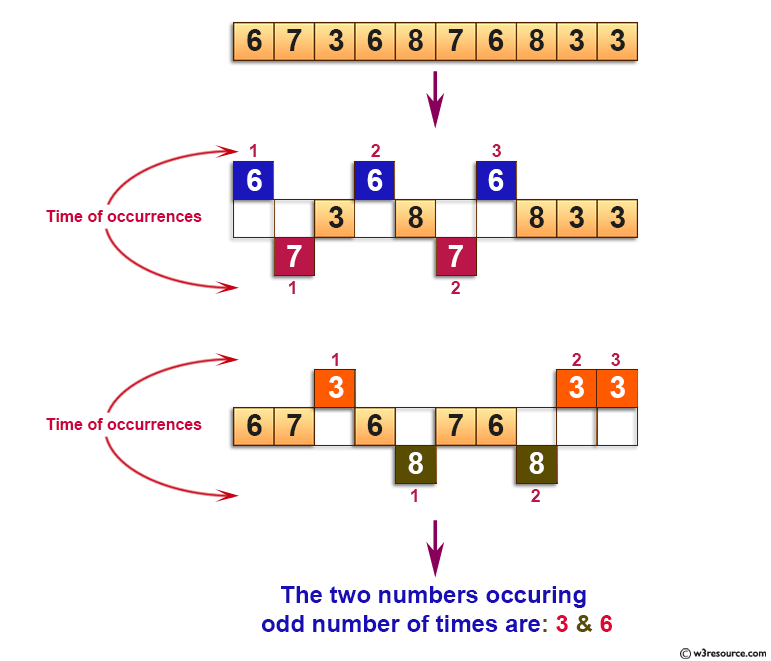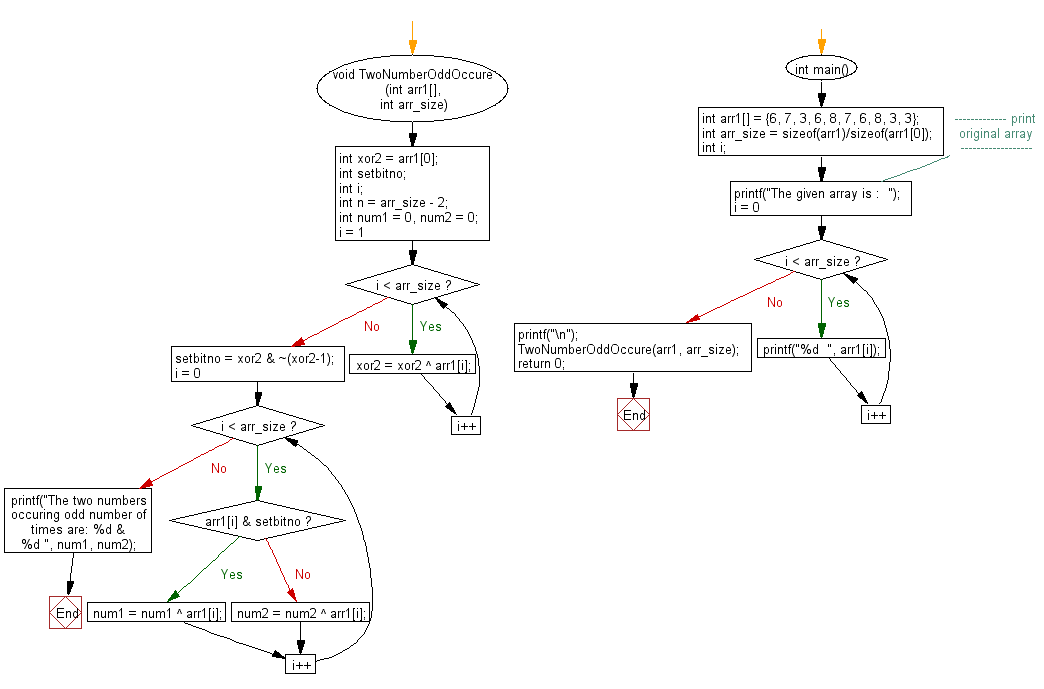﻿ C exercises: Find two numbers that occur odd number of times in an array - w3resource# C Exercises: Find two numbers that occur odd number of times in an array

## C Array: Exercise-70 with Solution

Write a program in C to find two numbers that occur odd number of times in an array.

Pictorial Presentation:Sample Solution:

C Code:

``````#include <stdio.h>

void TwoNumberOddOccure(int arr1[], int arr_size)
{
int xor2 = arr1;
int setbitno;
int i;
int n = arr_size - 2;
int num1 = 0, num2 = 0;
for(i = 1; i < arr_size; i++)
xor2 = xor2 ^ arr1[i];
setbitno = xor2 & ~(xor2-1);
for(i = 0; i < arr_size; i++)
{
if(arr1[i] & setbitno)
num1 = num1 ^ arr1[i];
else
num2 = num2 ^ arr1[i];
}
printf("The two numbers occuring odd number of times are: %d & %d ", num1, num2);
}

int main()
{
int arr1[] = {6, 7, 3, 6, 8, 7, 6, 8, 3, 3};
int arr_size = sizeof(arr1)/sizeof(arr1);
int i;
//------------- print original array ------------------
printf("The given array is :  ");
for(i = 0; i < arr_size; i++)
{
printf("%d  ", arr1[i]);
}
printf("\n");
//------------------------------------------------------
TwoNumberOddOccure(arr1, arr_size);
return 0;
}
```
```

Sample Output:

```The given array is :  6  7  3  6  8  7  6  8  3  3
The two numbers occuring odd number of times are: 3 & 6
```

Flowchart:C Programming Code Editor:

Improve this sample solution and post your code through Disqus.

What is the difficulty level of this exercise?

﻿